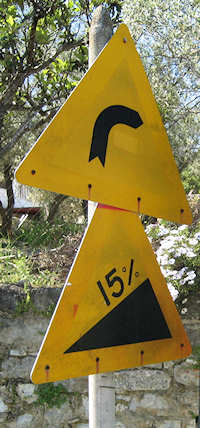Search IntMath
Close

450+ Math Lessons written by Math Professors and Teachers

5 Million+ Students Helped Each Year

1200+ Articles Written by Math Educators and Enthusiasts

Simplifying and Teaching Math for Over 23 Years

# Gradient (or slope) of a Line, and InclinationA 15% road gradient is equivalent to m = 0.15.

The gradient (also known as slope) of a line is defined as

"gradient"= text(vertical rise)/text(horizontal run

In the following diagram, the gradient of the line AB is given by: a/b

In general, for the line joining the points (x1, y1) and (x2, y2), we have:

(x_1,y_1)
(x_2,y_1)
(x_2,y_2)
y_2-y_1
x_2-x_1
"slope" = (y_2-y_1)/(x_2-x_1)

Slope of the line joining the points (x1, y1) and (x2, y2).

We can now write the formula for the slope of a line.

## Gradient of a Line Formula

We see from the diagram above, that the gradient (usually written m) is given by:

m=(y_2-y_1)/(x_2-x_1

## Interactive graph - slope of a line

You can explore the concept of slope of a line in the following interactive graph (it's not a fixed image).

Drag either point A (x1, y1) or point B (x2, y2) to investigate how the gradient formula works. The numbers will update as you interact with the graph.

Notice what happens to the sign (plus or minus) of the slope when point B is above or below A.

Slope = (y_2 - y_1)/(x_2 - x_1)

=(BC)/(AC)

You can move the graph up-down, left-right if you hold down the "Shift" key and then drag the graph.

If you get lost, you can always refresh the page.

### Example

Find the slope of the line joining the points (−4, −1) and (2, −5).

These are the points involved:

So the slope is:

m=(y_2-y_1)/(x_2-x_1

=(-5-(-1))/(2-(-4)

=(-4)/6

=-2/3

Note the slope is negative. The line is going "down hill" as we move left to right.

### Positive and Negative Slopes

In general, a positive slope indicates the value of the dependent variable (usually y) increases as we go left to right:

"slope " m " is positive"

The line has positive slope.

The dependent variable in the above graph is the y-value, while the independent variable is x.

A negative slope means that the value of the dependent variable (usually y) is decreasing as we go left to right:

"slope " m " is negative"

The line has negative slope.

## Inclination

We have a line with slope m and the angle that the line makes with the x-axis is α.

From trigonometry, we recall that the tan of angle α is given by:

tan\ alpha=text(opposite)/text(adjacent)

Now, since slope is also defined as opposite/adjacent, we have:

alpha
tan alpha = "opposite"/"adjacent" = m

Diagram illustrating tan α = m.

This gives us the result:

tan α = m

Then we can find angle α using

α = arctan m

(That is, α = tan-1 m)

This angle α is called the inclination of the line.

### Exercise 1

Find the inclination of the line with slope 2.

Here, tan α = 2, so

alpha=arctan(2)

=63.43^@

NOTE: The size of angle α is (by definition) only between 0° and 180°`.

### Exercise 2

Find the slope of the line with inclination α = 137°.

The situation is as follows:

So the slope is:

m = tan α

= tan 137°

= −0.933

Note that the slope is negative.

## Problem SolverThis tool combines the power of mathematical computation engine that excels at solving mathematical formulas with the power of GPT large language models to parse and generate natural language. This creates math problem solver thats more accurate than ChatGPT, more flexible than a calculator, and faster answers than a human tutor. Learn More.